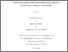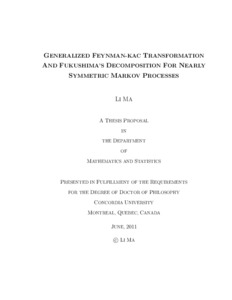Title:

# Generalized Feynman-Kac Transformation and Fukashima's Decomposition for Nearly Symmetric Markov Processes

Ma, Li (2011) Generalized Feynman-Kac Transformation and Fukashima's Decomposition for Nearly Symmetric Markov Processes. PhD thesis, Concordia University.Preview
Text (application/pdf)
Ma_PhD_F2011.pdf - Accepted Version
901kB

## Abstract

In this thesis, we study some problems about nearly symmetric Markov processes,which are associated with non-symmetric Dirichlet forms or semi-Dirichlet forms. For a Markov process (Xt, Px) associated with a non-symmetric Dirichlet form (E,D(E)) on L2(E;m), we study the strong continuity of the generalized Feynman- Kac semigroup (Pu
t )t≥0, which is defined by Pu t f(x) := Ex[eNu t f(Xt)], f ≥ 0 and t ≥ 0.Here u ∈ D(E), Nu t is the continuous additive functional of zero energy in the Fukushima’s decomposition. We give two sufficient conditions for (Pu
t )t≥0 to be strongly continuous.The first sufficient condition is that there exists a constant α0 ≥ 0 such that for any f ∈ D(E)b, Qu(f, f) ≥ −α0(f, f)m, where (Qu,D(E)b) is defined by Qu(f, g) := E(f, g) + E(u, fg), f,g∈ D(E)b := D(E) ∩ L∞(E;m). The second sufficient condition is that there exists a constant α0 ≥ 0 such that �Pu
t �2 ≤ eα0t, ∀t > 0. For a Markov process associated with a semi-Dirichlet form, we establish Fukushima’s
decomposition and give a transformation formula for local martingale additive functionals.

Divisions: Concordia University > Faculty of Arts and Science > Mathematics and Statistics Thesis (PhD) Ma, Li Concordia University Ph. D. Mathematics 29 June 2011 Sun, Wei Dirichlet forms, strong continuous, generalized Feynman-Kac semigroup, semi-Dirichlet forms, Fukushima's decomposition, local martingale additive functionals, zero energy, transformation formula. 7696 LI MA 22 Nov 2011 13:44 18 Jan 2018 17:31

## References:

[AFRS1995] S. Albeverio, R.Z. Fan, M. R¨ockner and W. Stannat: A remark on coercive forms and associated semigroups, Partial Differential Operators and Mathematical
Physics, Operator Theory Advances and Applications. 78, (1995), 1-8.
[AM1991] S. Albeverio and Z.M. Ma: Perturbation of Dirichlet forms-lower semiboundedness, closablility, and form cores. J. Funct. Anal. 99, (1991), 332-356.
[AM1992] S. Albeverio and Z.M. Ma: Additive functionals, nowhere Radon and Kato class smooth measures associated with Dirichlet forms. Osaka J. Math. 29, (1992),
247-265.
[C2007] C.Z. Chen: A note on perturbation of nonsymmetric Dirichlet forms by signed smooth measures. Acta Math. Scientia 27B, (2007), 219-224.
[CHM2010] C.Z. Chen, X.F. Han and L. Ma : Some new results about asymptotic properties of additive functionals of Brownian Motion. Acta Mathematica Scientia. 30 (6) (A), (2010), 1485-1494.
[CMS2007] C.Z. Chen, Z.M. Ma and W. Sun: On Girsanov and generalized Feynman-Kac transfromations for symmetric Markov processes. Infin. Dimens. Anal. Quantum
Probab. Relat. Top. 10, (2007), 141-163.
[CS2006] C.Z. Chen and W. Sun: Strong continuity of generalized Feynman-Kac semigroups: necessary and sufficient conditions. J. Funct. Anal. 237, (2006), 446-
465.
[CS2009] C.Z. Chen and W. Sun: Girsanov transformations for non-symmetric diffusions. Canad. J. Math. 61, (2009), 534-547.
[Z2005] Z.Q. Chen: On Feynman-Kac perturbation of symmetric Markov processes. Proceedings of Functional Analysis IX, Dubrovnik, Croatia. (2005), 39-43.
[CFKZ2008a] Z.Q. Chen, P.J. Fitzsimmons, K. Kuwae and T.S. Zhang: Stochastic calculus for symmetric Markov processes. Ann. Probab. 36, (2008), 931-970.
[CFKZ2008b] Z.Q. Chen, P.J. Fitzsimmons, K. Kuwae and T.S. Zhang: Perturbation of symmetric Markov Processes. Probab. Theory Relat. Fields. 140, (2008), 239-275.
[CFKZ2009] Z.Q. Chen, P.J. Fitzsimmons, K. Kuwae and T.S. Zhang: On general perturbations of symmetric Markov processes. J. Math. Pures et Appliqu´ees. 92, (2009), 363-374.
[CMR1994] Z.Q. Chen, Z.M. Ma and M. R¨ockner: Quasi-homeomorphisms of Dirichlet forms. Nagoya Math. J. 136, (1994), 1-15.
[CS2003] Z.Q. Chen, R.M. Song: Conditional gauge theorem for non-local Feynman-Kac transforms. Probab. Theory Relat. Fields. 125, (2003), 45-72.
[CZ2002] Z.Q. Chen and T.S. Zhang: Girsanov and Feynman-Kac type transformations for symmetric Markov processes. Ann. Inst. H. Poincar´e Probab. Statist. 38,(2002), 475-505.
[F2001] P.J. Fitzsimmons: On the quasi-regularity of semi-Dirichlet forms. Potential Anal. 15, (2001), 158-185.
[FK2004] P.J. Fitzsimmons and K. Kuwae: Nonsymmetric perturbations of symmetric Dirichlet forms. J. Funct. Anal. 208, (2004), 140-162.
[F1979] M. Fukushima: A decomposition of additive functionals of finite energy. Nagoya Math. J. 74, (1979), 137-168.
[FOT1994] M. Fukushima, Y. Oshima and M. Takeda: Dirichlet Forms and Symmetric Markov Processes. Walter de Gruyrer. Berlin, 1994.
[FU2010] M. Fukushima and T. Uemura: Jump-type Hunt processes generated by lower bounded semi-Dirichlet Forms. Preprint.
[GRSS1994] J. Glover, M. Rao, H. ˇSiki´c and R. Song: Quadratic forms corresponding to the generalized Schr¨odinger semigroups. J. Funct. Anal. 125, (1994), 358-378.
[HWY1992] S.W. He, J.G.Wang and J.A. Yan: Semimartingale Theory and Stochastic Calculus. Science Press. Beijing, 1992.
[HMS2006] Z.C. Hu, Z.M. Ma and W. Sun: Extensions of L´evy-Khintchine formula and Beurling-Deny formula in semi-Dirichlet forms setting. J. Funct. Anal. 239,
(2006), 179-213.
[HMS2010] Z.C. Hu, Z.M. Ma and W. Sun: On representations of non-symmetric Dirichlet forms. Potential Anal. 32, (2010), 101-131.
[HS2010] Z.C. Hu and W. Sun: Balayage of Semi-Dirichlet Forms. Preprint.
[K1987] J.H. Kim: Stochastic calculus related to non-symmetric Dirichlet forms, Osaka
J. Math. 24, (1987), 331-371.
[K2008] K. Kuwae: Maximum principles for subharmonic functions via local semi-Dirichlet forms. Can. J. Math. 60, (2008), 822-874.
[K2010] K. Kuwae: stochastic calculus over symmetric Markov processes without time reversal. Ann. Prob. 38(4), (2010), 1532-1569.
[MS2010a] L. Ma and W. Sun: On the generalized Feynman-Kac transformation for nearly symmetric Markov processes. Journal of Theoretical Probability, DOI:
10.1007/s10959-010-0318-3. 17
[MMS2011] L. Ma, Z.M. Ma and W. Sun: Fukushima’s decomposition for diffusions associated with semi-Dirichlet forms. submitted.
[MOR1995] Z.M. Ma, L. Overbeck and M. R¨ockner: Markov processes associated with semi-Dirichlet forms. Osaka J. Math. 32, (1995), 97-119.
[MR1992] Z.M. Ma and M. R¨ockner: Introduction to the Theory of (Non-Symmetric) Dirichlet Forms, Springer-Verlag, Berlin, 1992.
[MR1995] Z.M. Ma and M. R¨ockner: Markov processes associated with positivity preserving coercive forms. Can. J. Math. 47, (1995), 817-840.
[MS2010b] Z.M. Ma and W. Sun: Some topics on Dirichlet forms. Preprint, 2010.
[ORS1995] L. Overbeck, M. R¨ockner and B. Schmuland: An analytic approach to Fleming-Viot processes with interactive selection. Ann. Probab. 23, (1995), 1-36.
[O1988] Y. Oshima: Lecture on Dirichlet Spaces. Univ. Erlangen-N¨urnberg, 1988.
[P2005] P.E. Protter: Stochastic Integration and Differential Equations, Springer,Berlin Heidelberg New York, 2005.
[RS1995] M. R¨ockner and B. Schmuland: Quasi-regular Dirichlet forms: examples and counterexamples. Canadian Journal of Mathematics. 47(1), (1995), 165-200.
[V1991] Z. Vondracek.: An estimate for the L2−norm of a quasi continuous function with respect to a smooth measure. Arch. Math. 67, (1991), 408-414.
[T2001] T.S. Zhang: Generalized Feynman-Kac semigroups, associated quadratic forms and asymptotic properties. Potential Anal. 14, (2001), 387-408.
All items in Spectrum are protected by copyright, with all rights reserved. The use of items is governed by Spectrum's terms of access.

Repository Staff Only: item control pageResearch related to the current document (at the CORE website)
Back to top